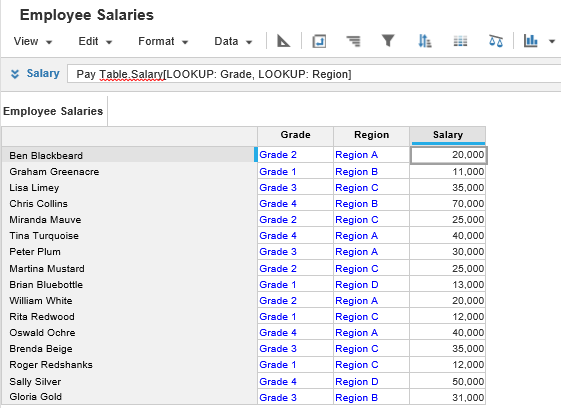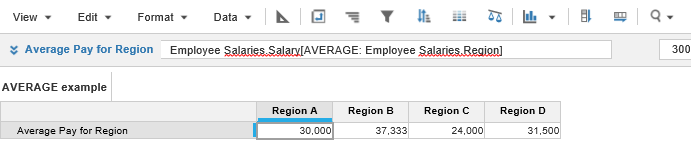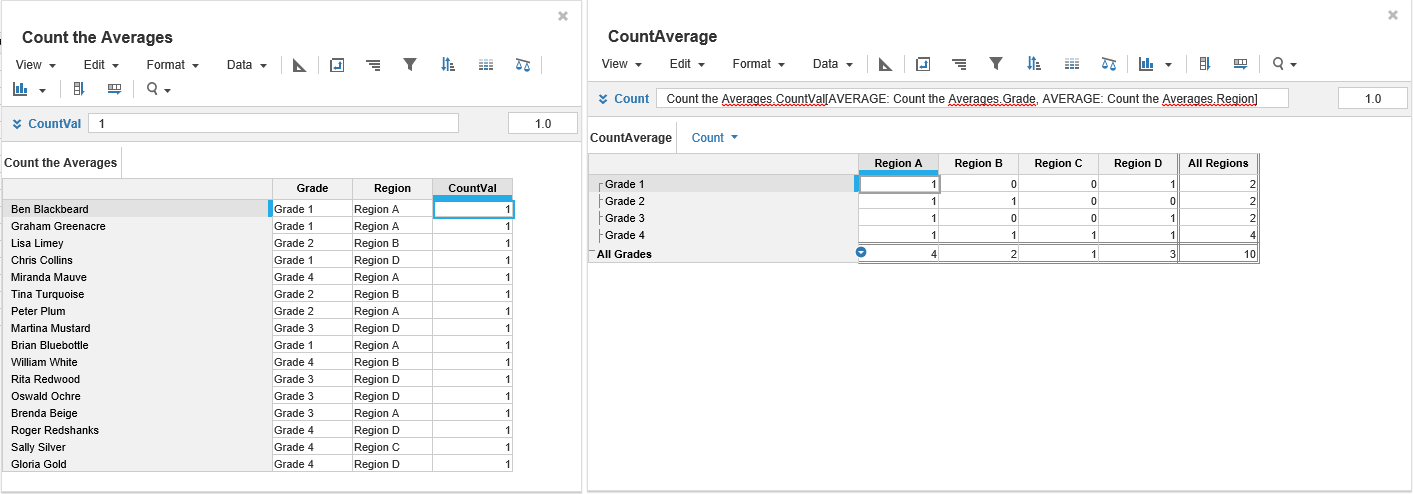1. Calculation functions
2. All Functions
3. Aggregation Functions
4. x[AVERAGE:y]Calculates the average for a range of values in a list.

## Syntax

x[AVERAGE: y]

where:

• x: value to average
• y: value specifying the list

## Format

Input Format Output Format

x: Number.

y: List

Number

## Arguments

The function uses the following arguments:

• x: Number: Numeric line item, property, or expression
• y: List

### Use AVERAGE with the Users list

You can reference the Users list with the AVERAGE function. However, you cannot reference specific users within the Users list as this is production data, which can change and make your formula invalid.

## Constraints

The function has the following constraints:

• Line items used for mappings must share a dimension with the source line item.
• Result line item must be number-formatted.
• Result line item must have each list used for mapping applied to it as a dimension.

## Example

In this example, an Employee Salaries source module shows staff Grade, Region, and Salary.The module has an Employees list as a dimension on Rows. The line items are on columns: Grade and Region is list-formatted on the Grades and Region lists respectively:

A results module has City Location as a dimension on Columns. We can then use AVERAGE in an Average Pay for Region, number-formatted, result line item to show the average employee salary paid in each region:

Employee Salaries.Salary[AVERAGE: Employee Salaries.Region]### Count Unique Values

The Count the Averages module allocates a CountVal of 1 to each Employee record.

The CountAverage module indicated where there is a unique combination of Grade and Region values, it does not count the number of occurrences. If there is no combination of a Grade value and a Region value, it returns 0.

For example, the Count the Averages table does not have a combination of Grade 1 and Region C, so the CountAverage table displays 0 at that intersection.

Count = Count the Averages.CountVal[AVERAGE: Count the Averages.Grade, AVERAGE: Count the Averages.Region]